# AP Calculus BC : Chain Rule and Implicit Differentiation

## Example Questions

1 3 Next →

### Example Question #21 : Chain Rule And Implicit Differentiation

Find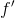: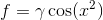, where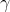is a constant.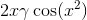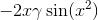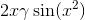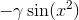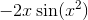Explanation:

The derivative of the function is equal to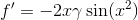and was found using the following rules: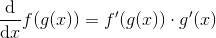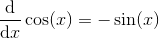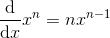The constant may seem intimidating, but we treat it as another constant!

### Example Question #61 : Computation Of Derivatives

Find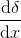from the following equation: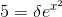, where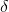is a function of x.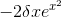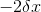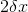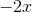Explanation:

To find the derivative ofwith respect to x, we must differentiate both sides of the equation with respect to x: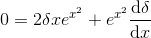The derivatives were found using the following rules: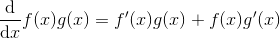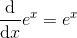Solving for, we get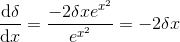Note that the chain rule was used because of the exponential and becauseis a function of x.

### Example Question #62 : Computation Of Derivatives

Find the first derivative of the following function: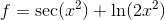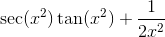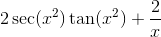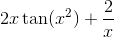Explanation:

The derivative of the function is equal to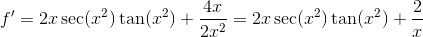and was found using the following rules: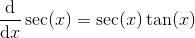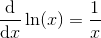Note that the chain rule was used on the secant function as well as the natural logarithm function.

### Example Question #63 : Computation Of Derivatives

Find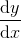: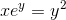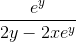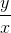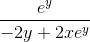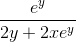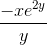Explanation:

To determine, we must take the derivative of both sides of the equation with respect to x: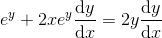The derivatives were found using the following rules:Rearranging and solving for, we get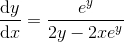### Example Question #64 : Computation Of Derivatives

A curve in the xy plane is given implictly by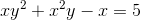.

Calculate the slope of the line tangent to the curve at the point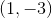.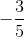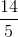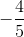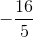Explanation:

Differentiate both sides with respect tousing the chain rule and the product rule as: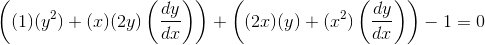Then solve for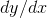as if it were our unknown: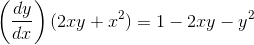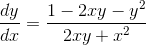.

Finally, evaluateat the pointto obtain the slope through that point: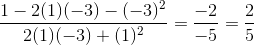.

### Example Question #26 : Chain Rule And Implicit Differentiation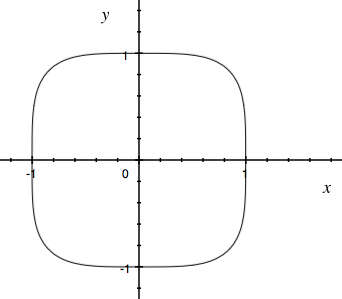squircle is a curve in the xy plane that appears like a rounded square, but whose points satisfy the following equation (analogous to the Pythagorean theorem for a circle)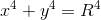where the constantis the "radius" of the squircle.

Using implicit differentiation, obtain an expression for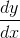as a function of bothand.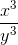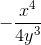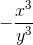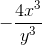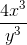Explanation:

Differentiate both sides of the equation with respect to, using the chain rule on the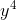term: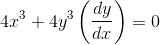Then solve foras if it were our unknown: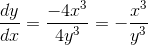.

Comparing this to the figure, our answer makes sense, because the slope of the squircle iswherever(as it crosses theaxis) and undefined (vertical) wherever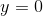(as it crosses theaxis). Lastly, we note that in the first quadrant (where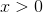and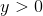), the slope of the squircle is negative, which is exactly what we observe in the figure.

1 3 Next →

### All AP Calculus BC Resources